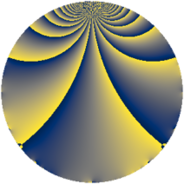# Properties

 Label 847.2.bLevel $847$ Weight $2$ Character orbit 847.b Rep. character $\chi_{847}(846,\cdot)$ Character field $\Q$ Dimension $64$ Newform subspaces $6$ Sturm bound $176$ Trace bound $4$

# Related objects

## Defining parameters

 Level: $$N$$ $$=$$ $$847 = 7 \cdot 11^{2}$$ Weight: $$k$$ $$=$$ $$2$$ Character orbit: $$[\chi]$$ $$=$$ 847.b (of order $$2$$ and degree $$1$$) Character conductor: $$\operatorname{cond}(\chi)$$ $$=$$ $$77$$ Character field: $$\Q$$ Newform subspaces: $$6$$ Sturm bound: $$176$$ Trace bound: $$4$$ Distinguishing $$T_p$$: $$2$$

## Dimensions

The following table gives the dimensions of various subspaces of $$M_{2}(847, [\chi])$$.

Total New Old
Modular forms 100 80 20
Cusp forms 76 64 12
Eisenstein series 24 16 8

## Trace form

 $$64q - 50q^{4} - 48q^{9} + O(q^{10})$$ $$64q - 50q^{4} - 48q^{9} - 2q^{14} - 20q^{15} + 26q^{16} + 8q^{23} - 40q^{25} + 70q^{36} + 24q^{37} + 16q^{42} + 28q^{49} - 24q^{53} - 82q^{56} - 52q^{58} - 68q^{60} + 86q^{64} - 68q^{67} + 28q^{70} - 20q^{71} + 76q^{78} + 8q^{81} + 4q^{86} + 76q^{91} - 20q^{92} + 20q^{93} + O(q^{100})$$

## Decomposition of $$S_{2}^{\mathrm{new}}(847, [\chi])$$ into newform subspaces

Label Dim. $$A$$ Field CM Traces $q$-expansion
$$a_2$$ $$a_3$$ $$a_5$$ $$a_7$$
847.2.b.a $$8$$ $$6.763$$ 8.0.$$\cdots$$.11 None $$0$$ $$0$$ $$0$$ $$0$$ $$q+(\beta _{2}+\beta _{4})q^{2}-\beta _{3}q^{3}+(-2+\beta _{6}+\cdots)q^{4}+\cdots$$
847.2.b.b $$8$$ $$6.763$$ 8.0.37515625.1 $$\Q(\sqrt{-7})$$ $$0$$ $$0$$ $$0$$ $$0$$ $$q+\beta _{1}q^{2}+(-1+\beta _{2})q^{4}+(-\beta _{1}+\beta _{4}+\cdots)q^{7}+\cdots$$
847.2.b.c $$8$$ $$6.763$$ 8.0.4956160000.2 None $$0$$ $$0$$ $$0$$ $$0$$ $$q+(\beta _{3}+\beta _{5})q^{2}+\beta _{6}q^{3}+(-1-\beta _{4}+\cdots)q^{4}+\cdots$$
847.2.b.d $$8$$ $$6.763$$ 8.0.$$\cdots$$.2 None $$0$$ $$0$$ $$0$$ $$0$$ $$q+\beta _{6}q^{2}-\beta _{1}q^{4}+\beta _{7}q^{5}+(-\beta _{3}-\beta _{5}+\cdots)q^{7}+\cdots$$
847.2.b.e $$16$$ $$6.763$$ $$\mathbb{Q}[x]/(x^{16} - \cdots)$$ None $$0$$ $$0$$ $$0$$ $$0$$ $$q+(-\beta _{3}+\beta _{10})q^{2}+(-\beta _{5}+\beta _{14}+\beta _{15})q^{3}+\cdots$$
847.2.b.f $$16$$ $$6.763$$ $$\mathbb{Q}[x]/(x^{16} + \cdots)$$ None $$0$$ $$0$$ $$0$$ $$0$$ $$q+(\beta _{1}+\beta _{5})q^{2}-\beta _{14}q^{3}+(1-\beta _{6}-\beta _{8}+\cdots)q^{4}+\cdots$$

## Decomposition of $$S_{2}^{\mathrm{old}}(847, [\chi])$$ into lower level spaces

$$S_{2}^{\mathrm{old}}(847, [\chi]) \cong$$ $$S_{2}^{\mathrm{new}}(77, [\chi])$$$$^{\oplus 2}$$